+

# Special Products of Binomials

Author: Sophia Tutorial
##### Description:

Expand a special product binomial.

(more)

Sophia’s self-paced online courses are a great way to save time and money as you earn credits eligible for transfer to many different colleges and universities.*

No credit card required

37 Sophia partners guarantee credit transfer.

299 Institutions have accepted or given pre-approval for credit transfer.

* The American Council on Education's College Credit Recommendation Service (ACE Credit®) has evaluated and recommended college credit for 32 of Sophia’s online courses. Many different colleges and universities consider ACE CREDIT recommendations in determining the applicability to their course and degree programs.

Tutorial
what's covered
1. FOIL Review
2. Square of a Binomial Sum
3. Square of a Binomial Difference
4. Difference of Squares

# 1. FOIL Review

Our special products of binomials come through by noticing how expressions are simplified in binomial multiplication. Binomial multiplication is often modeled through a process known as FOIL, which is an acronym that stands for First, Outside, Inside, Last. It describes an order to multiply terms that make up the two factors in binomial multiplication.

Below is an example of using FOIL to multiply two binomials: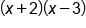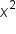Multiply first terms: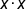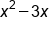Multiply outside terms: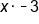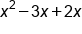Multiply inside terms: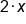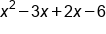Multiply last terms: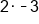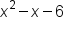Combine like terms

# 2. Square of a Binomial Sum

Take a look at what happens when we expand a binomial sum that is being squared: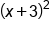Square of a binomial sum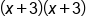Two factors of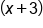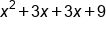FOIL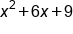Combine like terms

Note the relationship between the constant in our binomial sum (3), the coefficient of the x-term (6), and the constant term in the expanded trinomial (9). See if the relationship you spotted holds true in another example: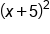Square of a binomial sum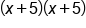Two factors of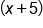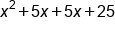FOIL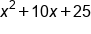Combine like terms

What is the relationship between 5, 10, and 25? If we take the constant from the binomial sum, 5, and double this number, we get the coefficient of x-term, 10. And if we square 5, we get 25, which is the constant term in the expanded trinomial. This holds true for all squares of binomial sums.

formula
Square of a Binomial Sum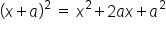# 3. Square of a Binomial Difference

Next, let's take a look at similar expressions, except these examples involve subtraction instead of addition: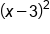Square of a binomial sum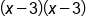Two factors of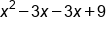FOIL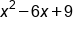Combine like terms

Note that the only distinction here is that 6x is –6x, because we subtracted 3 from x in the binomial. We still have +9 because –3 times –3 is a positive number. Let's take a look at another example: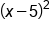Square of a binomial sum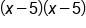Two factors of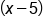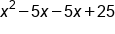FOIL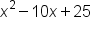Combine like terms

We can generalize this as the square of a binomial difference:

formula
Square of a Binomial Difference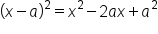The expanded form for the square of a binomial sum and the square of a binomial difference are also referred to as perfect square trinomials, because they consist of three terms, which can be simplified into an expression squared.

term to know
Perfect Square Trinomial
a polynomial with three terms, we can be simplified as a binomial squared,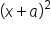or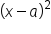# 4. Difference of Squares

So far we have discussed special products involving a binomial squared, where a is either positive or negative (which makes the distinction between addition/binomial sum, or subtraction/binomial difference. Now, we are going to consider the case where we have a binomial sum multiplied by a binomial difference.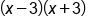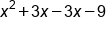FOIL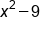Combine like terms

There are a couple of things to note in this example:

• The x-terms canceled, because the coefficients were opposites of each other.
• The constant term here is negative, because the product of a positive and negative number is always negative.
• The constant term is also a perfect square.

We can generalize this as a difference of squares.

formula
Difference of Squares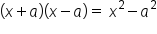term to know
Difference of Squares
Two squared terms separated by subtraction, x squared minus a squared, which can be expressed as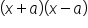summary
A foil review reminds us that FOIL is the acronym used to remember the way to multiply two binomials. It stands for First, Outside, Inside, Last. Recognizing special products of binomials can help make factoring and FOILing easier. The three special products are square of a binomial sum: (x+a)², square of a binomial difference: (x-a)², and the difference of squares: (x+a)(x-a). A perfect square trinomial is a polynomial with three terms that can be simplified as a binomial squared.

Terms to Know
Difference of Squares

two squared terms separated by subtraction, x^2 - a^2, which can be expressed as (x+a)•(x-a)

Perfect Square Trinomial

a polynomial with three terms, which can be simplified as a binomial squared, (x+a)^2

Formulas to Know
Difference of SquaresSquare of a Binomial DifferenceSquare of a Binomial SumRating